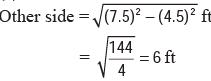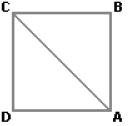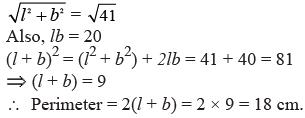Courses

# Olympiad Test: Elementary Mensuration-I -1

## 20 Questions MCQ Test Mathematics Olympiad Class 7 | Olympiad Test: Elementary Mensuration-I -1

Description
This mock test of Olympiad Test: Elementary Mensuration-I -1 for Class 7 helps you for every Class 7 entrance exam. This contains 20 Multiple Choice Questions for Class 7 Olympiad Test: Elementary Mensuration-I -1 (mcq) to study with solutions a complete question bank. The solved questions answers in this Olympiad Test: Elementary Mensuration-I -1 quiz give you a good mix of easy questions and tough questions. Class 7 students definitely take this Olympiad Test: Elementary Mensuration-I -1 exercise for a better result in the exam. You can find other Olympiad Test: Elementary Mensuration-I -1 extra questions, long questions & short questions for Class 7 on EduRev as well by searching above.
QUESTION: 1

### The ratio between the length and the breadth of a rectangular park is 3 : 2. If a man cycling along the boundary of the park at the speed of 12 km/hr completes one round in 8 minutes, then the area of the park (in sq. m) is:

Solution:

Perimeter = Distance covered in 8 min.
= (12000/60 × 8) = 1600 m
Let length = 3x metres and breadth
= 2x metres.
Then, 2(3x + 2x) = 1600 or x = 160.
∴ Length = 480 m and Breadth = 320 m.
∴ Area = (480 × 320) m2 = 153600 m2.

QUESTION: 2

### An error 2% in excess is made while measuring the side of a square. The percentage of error in the calculated area of the square is:

Solution:

100 cm is read as 102 cm.
∴ A1 = (100 × 100) cm2 and
A2 = (102 × 102) cm2.
(A2 – A1) = [(102)2 – (100)2]
= (102 + 100) × (102 – 100)
= 404 cm2
∴ Percentage error
= [404/(100 × 100) × 100]% = 4.04%

QUESTION: 3

### The ratio between the perimeter and the breadth of a rectangle is 5 : 1. If the area of the rectangle is 216 sq. cm, what is the length of the rectangle?

Solution:

Given
2(l + b)/b = 5/l
⇒ 2l + 2b = 5b
⇒ 3b = 2l
b = 2l/3
Then, Area = 216 cm2
⇒ l × b = 216
⇒ l × 2l/3 = 216
⇒ l2 = 324
⇒ l = 18 cm

QUESTION: 4

The percentage increase in the area of a rectangle, if each of its sides is increased by 20%, is:

Solution:

Let original length = x metres and original breadth = y metres.
Original area = (xy) m2.
New length = (120/100) × m = (6/5) × m
New breadth = (120/100) y m = (6/5) y m
New area = (6/5) × m × (6/5) y m
= (36/25)xy m2
The difference between the original area
= xy  and new area 36/25 xy is
= (36/25)xy – xy
= xy( 36/25 – 1)
= xy( 11/25) or (11/25)xy
∴ Increase %
= [(11/25)xy × 1/xy × 100]%
= 44%

QUESTION: 5

A rectangular park 60 m long and 40 m wide has two concrete crossroads running in the middle of the park and rest of the park has been used as a lawn. If the area of the lawn is 2109 sq. m, then what is the width of the road?

Solution:

Area of the park = (60 × 40) m2
= 2400 m2
Area of the lawn = 2109 m2.
∴ Area of the crossroads
= (2400 – 2109) m2 = 291 m2.
Let the width of the road be x metres. Then,
60x + 40x – x2 = 291
⇒ x2 – 100x + 291 = 0
⇒ (x – 97)(x – 3) = 0
⇒ x = 3

QUESTION: 6

The diagonal of the floor of a rectangular closet is 7.5 feet. The shorter side of the closet is 4.5 feet. What is the area of the closet in square feet?

Solution:∴ Area of closet = (6 × 4.5) sq. ft = 27 sq. ft.

QUESTION: 7

A towel, when bleached, was found to have lost 20% of its length and 10% of its breadth. The percentage of decrease in area is:

Solution:

Let original length = x
and original breadth = y
Decrease in area
= xy – [(80/100)x × (90/100)y]
= (7/25)xy
∴ Decrease %  = [(7/25)xy × 1/xy × 100]%
= 28%

QUESTION: 8

A man walked diagonally across a square plot. Approximately, what is the percent saved by not walking along the edges?

Solution:

Let the side of the square (ABCD) be x metres.
Then, AB + BC = 2x metres.AC = √2x = (1.41x) m.
Saving on 2x metres = (0.59x) m.
Saving % = (0.59x/2x) × 100%
= 30 %( approx)

QUESTION: 9

The diagonal of a rectangle is 41 cm and its area is 20 sq. cm. The perimeter of the rectangle must be:

Solution:

If l and b are length and breadth thenQUESTION: 10

What is the least number of squares tiles required to pave the floor of a room 15 m 17 cm long and 9 m 2 cm broad?

Solution:

Length of largest tile
= H.C.F. of 1517 cm and 902 cm = 41 cm.
Area of each tile = (41 × 41) cm2.
∴ Required number of tiles
= (1517 × 902)/(41 × 41) = 814

QUESTION: 11

The difference between the length and breadth of a rectangle is 23 m. If its perimeter is 206 m, then its area is:

Solution:

We have: (l – b) = 23 and 2(l + b) = 206 or
(l + b) = 103.
where, l =  length , b = breath
Solving these two equations, we get:
l = 63 and b = 40.
∴ Area = (l × b) = (63 × 40) m2
= 2520 m2.

QUESTION: 12

The length of a rectangle is halved, while its breadth is tripled. What is the percentage change in area?

Solution:

Let original length = x
and original breadth = y
Original area = xy.
New length = x/2
New breadth = 3y.
New area = (x/2 × 3y) = (3/2)xy
∴ Increase %  = (1/2)xy × (1/xy) × 100%
= 50%

QUESTION: 13

The length of a rectangular plot is 20 metres more than its breadth. If the cost of fencing the plot @ 26.50 per metre is Rs 5300, what is the length of the plot in metres?

Solution:

Let breadth = x metres.
Then, length = (x + 20) metres.
Perimeter = (5300/26.50) m = 200 m
∴ 2[(x + 20) + x] = 200
⇒ 2x + 20 = 100
⇒ 2x = 80
⇒ x = 40
Hence, length = x + 20 = 60 m.

QUESTION: 14

A rectangular field is to be fenced on three sides leaving a side of 20 feet uncovered. If the area of the field is 680 sq. feet, how many feet of fencing will be required?

Solution:

We have: l = 20 ft and lb = 680 sq. ft.
So, b = 34 ft.
∴ Length of fencing = (l + 2b)
= (20 + 68) ft = 88 ft.

QUESTION: 15

A tank is 25 m long, 12 m wide and 6 m deep. The cost of plastering its walls and bottom at 75 paise per sq. m, is:

Solution:

Area to be plastered
= [2(l + b) × h] + (l × b)
= {[2(25 + 12) × 6] + (25 × 12)} m2
= (444 + 300) m2
= 744 m2.
∴ Cost of plastering = Rs (744 x 75/100)
= Rs 558

QUESTION: 16

The area of playground is 1600 m2. What is the perimeter?
I. It is a perfect square playground.
II. It costs Rs 3200 to put a fence around the playground at the rate of Rs 20 per metre.

Solution:

Area = 1600 m2.
I. Side = 1600 m = 40 m. So, perimeter
= (40 × 4) m = 160 m.
∴ I alone gives the answer.
II. Perimeter = Total cost = 3200 m
= 160 m
Cost per metre = 20
∴ II alone gives the answer.
∴ Correct answer is (c).

QUESTION: 17

The area of a rectangle is equal to the area of right-angles triangle. What is the length of the rectangle?
I. The base of the triangle is 40 cm.
II. The height of the triangle is 50 cm.

Solution:

Given: Area of rectangle
= Area of a right-angle triangle.
⇒ l x b = ½ x B x H
I gives, B = 40 cm.
II gives, H = 50 cm.
Thus, to find l, we need b also, which is not given.
∴ Given data is not sufficient to give the answer.
∴ Correct answer is (d).

QUESTION: 18

What is the height of the triangle?
I. The area of the triangle is 20 times its base.
II. The perimeter of the triangle is equal to the perimeter of a square of side 10 cm.

Solution:

I. A = 20 x B ½ x B x H = 20 x B
⇒ H = 40.
∴ I alone gives the answer.
II gives the perimeter of the triangle = 40 cm.
This does not give the height of the triangle.
∴ Correct answer is (a).

QUESTION: 19

What will be the cost of painting the inner walls of a room if the rate of painting is Rs 20 per square foot?
I. Circumference of the floor is 44 feet.
II. The height of the wall of the room is 12 feet.

Solution:

I gives, 2 πR = 44
II gives, H = 12.
∴ A = 2 πRH = (4 × 12)
Cost of painting = Rs (44 × 12 × 20)
Thus, I and II together give the answer.
∴ Correct answer is (d).

QUESTION: 20

What is the area of the hall?
I. Material cost of flooring per square metre is Rs 2.50.
II. Labour cost of flooring the hall is Rs 3500.
III. Total cost of flooring the hall is Rs 14,500.

Solution:

I. Material cost = Rs 2.50 per m2
II. Labour cost = Rs 3500
III. Total cost = Rs 14,500
Let the area be A sq. metres.
∴ Material cost = Rs (14500 – 3500)
= Rs 11,000
∴ 5A/2 = 11000
A = (11000 × 2)/5 = 4400 m2
Thus, all I, II and III are needed to get the answer.
∴ Correct answer is (c).